## 4Fe (s) + 3 O2 (g) → 2 Fe2O3 (s) A. What is the oxidation number for each item in this equation? B. What is being oxidized?

Question

4Fe (s) + 3 O2 (g) → 2 Fe2O3 (s)
A. What is the oxidation number for each item in this equation?
B. What is being oxidized?
C. What is being reduced?
D. What is the oxidizing agent?
E. What is the reducing agent?

in progress 0
2 months 2021-07-24T18:13:56+00:00 1 Answers 5 views 0

1. Explanation:

The number acquired by an element after the lose or gain of an electron is called oxidation number.

For example,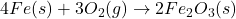Here, oxidation number of Fe(s) is 0 and Fe in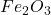is +3.

Oxidation number of O in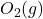is 0 as it is present in its elemental state.

The oxidation number of O inis calculated as follows.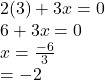Hence,  oxidation number of O inis -2.

• The loss of electrons by an element or substance is called oxidation. Here, electrons are being lost by Fe(s) as an increase in oxidation state is occurring. So, Fe(s) is oxidized.
• The gain of electrons by an element or substance is called reduction. Here, electrons are being added to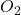as a decrease in its oxidation state is occurring. So,is reduced.
• An element or compound which is being reduced is called oxidizing agent. Here,is the oxidizing agent.
• An element or compound which is being oxidized is called reducing agent. Here, Fe(s) is the reducing agent.